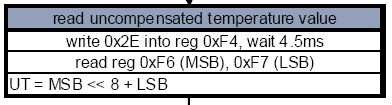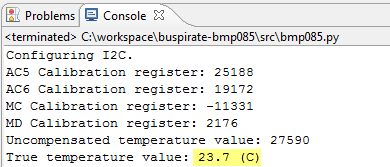## Bus pirate: reading BMP085 temperature

This post continues upon the last Bus Pirate post. In that post I was able to read a register from a BMP085 pressure chip.
In this post I will use the pyBusPirate python library to read the BMP085 temperature value (pyBusPirate allows you to script actions to the bus pirate)

Let’s start by reading the uncompensated (raw) temperature value, as described in the datasheet:Here’s my raw temperature read out function:

```    def read_temp_raw(self):
"""
Reads raw temperature from BMP085 pressure chip using bus pirate.
"""
# Start new temperature measurement
self.control_register(0xf4, 0x2e)

# Read MSB and LSB from registers
msb = self.get_register(0xf6)
lsb = self.get_register(0xf7)

# Calculate uncompensated temperature
self.UT = (msb << 8) + lsb
print "Uncompensated temperature value: %s" % self.UT
```

control_register() and get_register() are little helper function I wrote to control and read a register. The other interesting part in this code is the bitshifting to calculate the uncompensated temperature value. A MSB (Most Significant Bit) and LSB (Least Significant Bit) are read from the registers and “combined” into one value. This is done using bitshifting. The raw temperature is supposed to be a 16bit c-type short. 16 bits aka 2 bytes. So let’s see what happens here:

UT = 16bit (2 bytes)
MSB = 8bit (1 byte)
LSB = 8bit (1 byte)

MSB << 8 moves the MSB 8 bit positions to the left. For example: UT = 0000000000000000 MSB = 11001010 LSB = 00001100 MSB << 8 = 1100101000000000 (see how the bits moved?) MSB << 8 + LSB = 1100101000001100 = UT To calculate the true temperature value, the following formula is used:Here’s the relevant part of the code:

```    def calculate_temp(self):
"""
Calculates the true temperature value.
"""
x1 = (self.UT - self.AC6) * self.AC5 >> 15
x2 = (self.MC << 11) / (x1 + self.MD)
b5 = x1 + x2
temp = (b5 + 8) >> 4
temp = str(temp)
print "True temperature value: %s.%s (C)" % (temp[0:2], temp)
```

Please note that the calibration registers have been retrieved earlier on in the code, using this piece of code:

```    def get_calibration(self):
"""
Reads calibration values from BMP085 registers.
"""
#self.i2c_write_data([0xee, 0xb2])
msb = self.get_register(0xb2)
lsb = self.get_register(0xb3)
self.AC5 = (msb << 8) + lsb
print "AC5 Calibration register:", self.AC5

msb = self.get_register(0xb4)
lsb = self.get_register(0xb5)
self.AC6 = (msb << 8) + lsb
print "AC6 Calibration register:", self.AC6

msb = self.get_register(0xbc)
lsb = self.get_register(0xbd)
self.MC = (msb << 8) + lsb
print "MC Calibration register:", self.MC

msb = self.get_register(0xbe)
lsb = self.get_register(0xbf)
self.MD = (msb << 8) + lsb
print "MD Calibration register:", self.MD
```

The same type of bitshifting is used for the MSB and LSB.
So let’s see how the script works:Succes! The complete code including modified pyBusPirate code to work on Windows is available on my github page.

You can leave a response, or trackback from your own site.

### 3 Responses to “Bus pirate: reading BMP085 temperature”

1.Daniel O'Connor says:

The print statement would be better written..

print “True temperature value: %.1f (C)” % (temp / 100.0)
(delete temp = str(temp))

Also I used this idiom for reading register values from it using the Bus Pirate python lib..

res = reduce(lambda x, y: x + y, i2c.get_bytes(0x77, 0xaa, 22))
(self.ac1, self.ac2, self.ac3, self.ac4, self.ac5, self.ac6, self.b1, self.b2, self.mb, self.mc, self.md) = struct.unpack(‘>HHHhhhHHHHH’, res)

2. […] Maarten Damen’s Bus Pirate reading the BMP085 Temperature […]

3. […] Maarten Damen’s Bus Pirate reading the BMP085 Temperature (site down at the time of writing this) […]